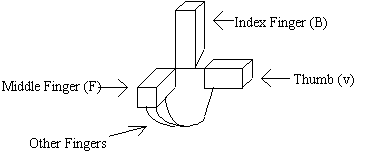Lorentz Force Law

The Lorentz Force Law can be used to describe the effects of a charged particle moving in a constant magnetic field. The simplest form of this law is given by the scalar equation

F = QvB

• F is the force acting on the particle (vector)
• v is velocity of particle (vector)
• Q is charge of particle (scalar)
• B is magnetic field (vector)

NOTE: this case is for v and B perpendicular to each other otherwise use F = QvB(sin(x)) where x is the angle between v and B. When v and B are perpendicular x=90 deg. so sin(x)=1.

The right hand rule comes into play here to figure out which way the force is acting.

Right Hand Rule

For a POSITIVLY charged particle moving (velocity v) in a magnetic field (field B) the direction of the resultant force (force F) can be found by:

• 1) THUMB of right hand in direction of VELOCITY, v (first vector)
• 2) INDEX FINGER of r.h. in direction of FIELD, B (second vector)
• 3) Your PALM (or middle finger if you like) now points in the direction of the FORCE, F (final vector)
• The force will ALWAYS be perpendicular to the PLANE of the vectors v and B, no matter what the angle between v and B is. Just pretend the following picture is of your right hand: